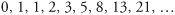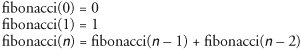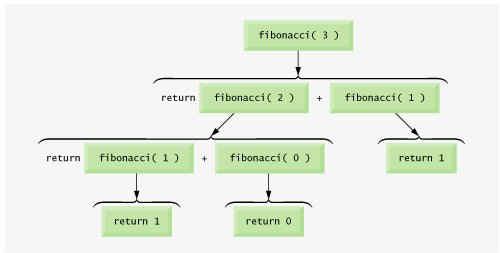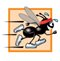# Example Using Recursion: Fibonacci Series

### Example Using Recursion Fibonacci Series

The Fibonacci series,begins with 0 and 1 and has the property that each subsequent Fibonacci number is the sum of the previous two Fibonacci numbers. This series occurs in nature and, in particular, describes a form of spiral. The ratio of successive Fibonacci numbers converges on a constant value of 1.618..., a number that has been called the golden ratio or the golden mean. Humans tend to find the golden mean aesthetically pleasing. Architects often design windows, rooms and buildings whose length and width are in the ratio of the golden mean. Postcards are often designed with a golden-mean length-to-width ratio.

The Fibonacci series may be defined recursively as follows:Note that there are two base cases for the Fibonacci calculation: fibonacci(0) is defined to be 0, and fibonacci(1) is defined to be 1. The program in Fig. 15.5 calculates the ith Fibonacci number recursively, using method fibonacci (lines 713). Method displayFibonacci (lines 1520) tests fibonacci, displaying the Fibonacci values of 010. The variable counter created in the for header in line 17 indicates which Fibonacci number to calculate for each iteration of the for statement. The value of counter is stored in variable number (line 7) for each call to method fibonacci. Fibonacci numbers tend to become large quickly. Therefore, we use type long as the parameter type and the return type of method fibonacci. Figure 15.6 calls method displayFibonacci (line 9) to calculate the Fibonacci values.

Figure 15.5. Fibonacci numbers generated with a recursive method.

 ``` 1 // Fig. 15.5: FibonacciCalculator.java 2 // Recursive fibonacci method. 3 4 public class FibonacciCalculator 5 { 6 // recursive declaration of method fibonacci 7 public long fibonacci( long number ) 8 { 9 if ( ( number == 0 ) || ( number == 1 ) ) // base cases 10 return number; 11 else // recursion step 12 return fibonacci( number - 1 ) + fibonacci( number - 2 ); 13 } // end method fibonacci 14 15 public void displayFibonacci() 16 { 17 for ( int counter = 0; counter <= 10; counter++ ) 18 System.out.printf( "Fibonacci of %d is: %d ", counter, 19 fibonacci( counter ) ); 20 } // end method displayFibonacci 21 } // end class FibonacciCalculator ```

Figure 15.6. Testing the fibonacci method.

(This item is displayed on page 751 in the print version)

``` 1 // Fig. 15.6: FibonacciTest.java
2 // Testing the recursive fibonacci method.
3
4 public class FibonacciTest
5 {
6 public static void main( String args[] )
7 {
8 FibonacciCalculator fibonacciCalculator = new FibonacciCalculator();
9 fibonacciCalculator.displayFibonacci();
10 } // end main
11 } // end class FibonacciTest
```

 ```Fibonacci of 0 is: 0 Fibonacci of 1 is: 1 Fibonacci of 2 is: 1 Fibonacci of 3 is: 2 Fibonacci of 4 is: 3 Fibonacci of 5 is: 5 Fibonacci of 6 is: 8 Fibonacci of 7 is: 13 Fibonacci of 8 is: 21 Fibonacci of 9 is: 34 Fibonacci of 10 is: 55 ```

The call to method fibonacci (line 19 of Fig. 15.5) from displayFibonacci is not a recursive call, but all subsequent calls to fibonacci performed from the body of fibonacci (line 12 of Fig. 15.5) are recursive, because at that point the calls are initiated by method fibonacci itself. Each time fibonacci is called, it immediately tests for the base casesnumber equal to 0 or number equal to 1 (line 9). If this condition is true, fibonacci simply returns number because fibonacci( 0 ) is 0, and fibonacci( 1 ) is 1. Interestingly, if number is greater than 1, the recursion step generates two recursive calls (line 12), each for a slightly simpler problem than the original call to fibonacci.

Figure 15.7 shows how method fibonacci evaluates fibonacci( 3 ). Note that at the bottom of the figure, we are left with the values 1, 0 and 1the results of evaluating the base cases. The first two return values (from left to right), 1 and 0, are returned as the values for the calls fibonacci( 1 ) and fibonacci( 0 ). The sum 1 plus 0 is returned as the value of fibonacci( 2 ). This is added to the result (1) of the call to fibonacci( 1 ), producing the value 2. This final value is then returned as the value of fibonacci( 3 ).

Figure 15.7. Set of recursive calls for fibonacci ( 3 ).

(This item is displayed on page 752 in the print version)Figure 15.7 raises some interesting issues about the order in which Java compilers evaluate the operands of operators. This order is different from the order in which operators are applied to their operandsnamely, the order dictated by the rules of operator precedence. From Figure 15.7, it appears that while fibonacci( 3 ) is being evaluated, two recursive calls will be madefibonacci( 2 ) and fibonacci( 1 ). But in what order will these calls be made? The Java language specifies that the order of evaluation of the operands is from left to right. Thus, the call fibonacci( 2 ) is made first and the call fibonacci( 1 ) is made second.

A word of caution is in order about recursive programs like the one we use here to generate Fibonacci numbers. Each invocation of the fibonacci method that does not match one of the base cases (0 or 1) results in two more recursive calls to the fibonacci method. Hence, this set of recursive calls rapidly gets out of hand. Calculating the Fibonacci value of 20 with the program in Fig. 15.5 requires 21,891 calls to the fibonacci method; calculating the Fibonacci value of 30 requires 2,692,537 calls! As you try to calculate larger Fibonacci values, you will notice that each consecutive Fibonacci number you use the application to calculate results in a substantial increase in calculation time and in the number of calls to the fibonacci method. For example, the Fibonacci value of 31 requires 4,356,617 calls, and the Fibonacci value of 32 requires 7,049,155 calls! As you can see, the number of calls to fibonacci increases quickly1,664,080 additional calls between Fibonacci values of 30 and 31 and 2,692,538 additional calls between Fibonacci values of 31 and 32! The difference in the number of calls made between Fibonacci values of 31 and 32 is more than 1.5 times the number of calls for Fibonacci values between 30 and 31. Problems of this nature can humble even the world's most powerful computers. [Note: In the field of complexity theory, computer scientists study how hard algorithms work to complete their tasks. Complexity issues are discussed in detail in the upper-level computer science curriculum course generally called "Algorithms." We introduce various complexity issues in Chapter 16, Searching and Sorting.] In the exercises, you will be asked to enhance the Fibonacci program of Fig. 15.5 such that it calculates the approximate amount of time required to perform the calculation. For this purpose, you will call static System method currentTimeMillis, which takes no arguments and returns the computer's current time in milliseconds.

Performance Tip 15.1Avoid Fibonacci-style recursive programs, because they result in an exponential "explosion" of method calls.Java How to Program (6th Edition) (How to Program (Deitel))
ISBN: 0131483986
EAN: 2147483647
Year: 2003
Pages: 615